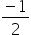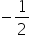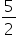Mathematics
Easy

Question

# Find the 11th term of the Arithmetic Sequences : -3 ,, 2………

## 10252223Hint:

## The correct answer is: 22

### The given sequence is -3,, 2, ...  The first term of the progression is a = -3Common difference is the fixed difference between the consecutive numbers of the sequence. We have to add the common difference to the preceding term, to get the next term. It can be negative or positive number. It can also have value zero.The common difference isThe formula for nth term of a arithmetic progression is given as follows:an = a + (n – 1)dWe will substitute n = 11 to find the 11th term.a11 = -3 + (11 - 1)= -3 + 25= -22So, the 11th term is 22.

For such questions, we should know the formula to find any number of the terms.

### Related Questions to study#### With Turito Foundation.#### Get an Expert Advice From Turito.